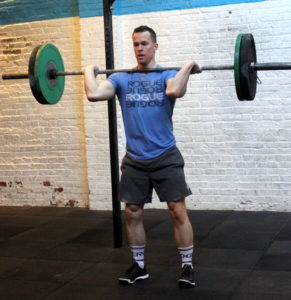## Thursday, September 26, 2019

### Thursday, September 26, 2019as many rounds as possible in 12 minutes:
12x front squat @ 115/75-lbs.
9x front rack weighted lunge
6x push press

### 1 Comment

1.–Scores–
Hari P.: 5 rounds + 1x lunge (105-lbs.)
Borja G.: 5 rounds + 6x front squat (85-lbs.)
Al C.: 4 rounds + 8x front squat (105-lbs.)
Aaron B.: 4 rounds + 6x front squat (80-lbs.)
Andy G.: 3 rounds + 6x front squat (95-lbs., 30-lb. db push press)
Tommy W.: 5 rounds + 8x front squat (75-lbs.)
Anu B.: 4 rounds + 1x front squat (85-lbs.)
Jin D.: 5 rounds + 6x front squat (55-lbs.)
Jason S.: 4 rounds + 4x lunge (75-lbs.)
David C.: 5 rounds + 6x front squat (75-lbs., 30-lb. dbs; sub: db lunge, db push press)
John S.: 5 rounds + 6x front squat (85-lbs.; sub: back rack lunge)
Francisco L.: 4 rounds + 9x lunge (85-lbs.)
Drew P.: 5 rounds+ 4x lunge (95-lbs.)
Will O.: 5 rounds + 2x front squat (85-lbs.)
Ralph B.: 4 rounds + 3x deadlift (95-lbs.; sub: deadlift, hang power clean)
Tara S.: 4 rounds + 9x lunge
JP S.: 5 round s+ 5x front squat (65-lbs.; sub: wgted lunge/12x bw lunge, alt rounds)
Michael Fo.: 4 rounds (75-lbs.)
John N.: 3 rounds + 2x lunge (65-lbs.)
John La.: 6 rounds (25-lb. db, 20-lb. dbs; sub: wgted glute bridge, steated muscle clean, seated shoulder press)
Diana D.: 4 rounds + 12x front squat (45-lbs.)
Matt M.: 5 rounds + 5x front squat (95-lbs.)
Patrick J.: 5 rounds + 7x lunge (75-lbs.)
Andrew Z.: 4 rounds + 2x push press (105-lbs.)
Gabriel F.: 5 rounds + 2x lunge (65-lbs.)
Henry T.: 5 round s+ 2x front squat (65-lbs.)
Simon B.: 3 rounds + 6x lunge (75-lbs.)
Adriana B.: 4 rounds + 7x lunge (55-lbs.)
Allie R.: 5 rounds + 6x squat (55-lbs.)
Lisa C.: 5 rounds + 11x front squat (45-lbs.)
Sergio L.: 4 rounds + 4x front squat (75-lbs.)
Yao Z.: 4 rounds + 2x push press (75-lbs.)
Zach D.: 5 rounds + 4x lunge
Chris T.: 4 rounds + 6x lunge (95-lbs.)
Dan C.: 4 rounds + 6x front squat (85-lbs.)
Chris P.: 3 rounds + 2x front squat (75-lbs.; sub: deadlift for lunge)
Joya TD.: 5 rounds + 11x front squat (65-lbs.)
Alli P.: 5 rounds + 1x lunge (65-lbs.)
Anna Go.: 3 rounds + 6x lunge (65-lbs.)
Andrew B.: 6 rounds + 4x lunge (55-lbs.; sub: deadlift for front squat)
Tarek K.: 5 rounds (65-lbs.; sub: no front squat final round)
Josh M.: 4 rounds + 2x front squat (95-lbs.)
Seth C.: 5 rounds + 9x front squat (75-lbs.)
Rafa C.: 3 rounds + 7x front squat (75-lbs.)
Julian M.: 3 rounds + 3x push press (25-lb. dbs)
Earl: 5 rounds (75-lbs.)
Dan D.: 5 rounds + 4x front squat (65-lbs.)
Mark Co.: 5 rounds + 5x front squat (95-lbs.)
Mark Ab.: 5 rounds + 6x front squat (sub: 45-lb. single-arm db push press)
Charlie K.: 5 rounds + 7x front squat (85-lbs.; sub: 45-lb. single-arm db push press)
Lily S.: 4 rounds + 1x push press (55-lbs.)
Adrienne V.: 5 rounds + 12x front squat (55-lbs.)
Terry A.: 3 rounds + 9x lunge (25-lbs.)
Seth B.: 4 rounds + 12x front squat (
David B.: 3 rounds + 1x lunge (55-lbs.; sub: 200m run after each run)